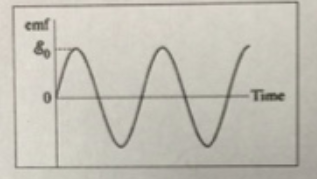# Problem: A generator has a coil of wire rotating in a magnetic field. If the rotation rate increases, how is the maximum output voltage of the generator affected?a) increasesb) decreasesc) stays the samed) varies sinusoidally

###### FREE Expert Solution

The operation of a generator depends on Faraday's law of electromagnetic induction.

According to this law, the induced emf ina  coil depends on the change in the magnetic flux as:

$\overline{){\mathbf{\epsilon }}{\mathbf{=}}{\mathbf{-}}\frac{\mathbf{d}\mathbf{\varphi }}{\mathbf{d}\mathbf{t}}}$

83% (431 ratings)###### Problem Details

A generator has a coil of wire rotating in a magnetic field. If the rotation rate increases, how is the maximum output voltage of the generator affected?a) increases

b) decreases

c) stays the same

d) varies sinusoidally